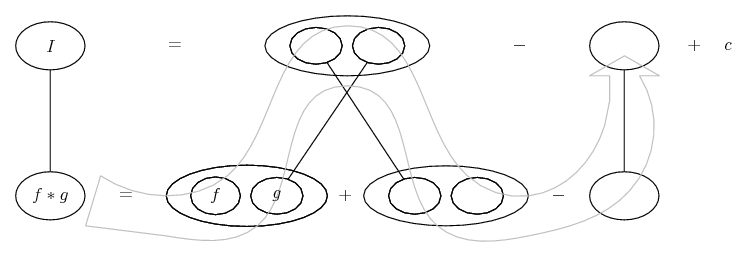# Thread: intergrals

1. ## intergrals

intergral of (x^3)(e^x^2) dx
I tried to do it with the formula intergral uv = uv - intergral v(du) and my answer come to 1/2(x^2)(e^x^2) - 3/4(e^x^2) + c but in the book says

1/2(x^2)(e^x^2) - 1/2(e^x^2) +c

2.Originally Posted by homerbintergral of (x^3)(e^x^2) dx
I tried to do it with the formula intergral uv = uv - intergral v(du) and my answer come to 1/2(x^2)(e^x^2) - 3/4(e^x^2) + c but in the book says

1/2(x^2)(e^x^2) - 1/2(e^x^2) +c
integrate &#40;x&#94;3&#41;&#40;e&#94;x&#94;2&#41; - Wolfram|Alpha

Click on Show steps.

3. Just in case a picture also helps...... where (key in spoiler) ...

Spoiler:... is the chain rule. Straight continuous lines differentiate downwards (integrate up) with respect to the main variable (in this case x), and the straight dashed line similarly but with respect to the dashed balloon expression (the inner function of the composite which is subject to the chain rule).

But this is wrapped inside the legs-uncrossed version of...... the product rule, where, again, straight continuous lines are differentiating downwards with respect to x.... is lazy integration by parts, doing without u and v.

_________________________________________

Don't integrate - balloontegrate!

Balloon Calculus; standard integrals, derivatives and methods

Balloon Calculus Drawing with LaTeX and Asymptote!

4. I admit that I don't really understand the diagram that tom made. I can show you have to do it by parts, though, if you insist on using that (though WolframAlpha's method is preferable.)

$\displaystyle \displaystyle \int p dq = pq - \int qdp$

Using $\displaystyle p = x^2 \implies dp = 2xdx$ and $\displaystyle \displaystyle dq = xe^{x^2}dx \implies q = \frac{1}{2}e^{x^2}$ (Use WolframAllpha's substitution $\displaystyle u = x^2$ here.)

So we get
$\displaystyle \displaystyle \int x^3e^{x^2}dx = \frac{1}{2}x^2e^{x^2} - \int xe^{x^2}dx$

The last integral is the same you used to get q, so...
$\displaystyle \displaystyle \int x^3e^{x^2}dx = \frac{1}{2}x^2e^{x^2} - \int xe^{x^2}dx = \frac{1}{2}x^2e^{x^2} - \frac{1}{2}e^{x^2} = \frac{1}{2}(x^2 - 1)e^{x^2}$

(plus an arbitrary constant, of course.)

-Dan

5.Originally Posted by topsquarkI admit that I don't really understand the diagram that tom made.
Oh dear, that's always embarassing! However, does it help if I zoom out of the chain rule shape, thus...Agreed, so far? Now the whole point of integration by parts, surely, is to treat the integrand as one 'fork' of a product-rule differentiation. So fill in the rest of this product rule process (where differentiation is downwards, anti-differentiation up)...Now the only problem is the whole derivative is unequal to the integrand. So fix that, and finish...Embedding the chain-rule shape into this diagram was just to help navigate the integration of x e^(x^2) without needing a substitution.

By the way, I don't see how you thought Wolfram's was any different to yours, or mine for that matter. We're all working backwards through the product rule and also the chain rule on the way.6. Don't call me Shirley.

7. Thanks tom. I get it now. That's a pretty neat construction.-Dan

#### Search Tags

intergrals